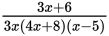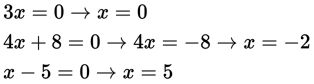# SAT Math Multiple Choice Question 893: Answer and Explanation

### Test Information

Question: 893

8. For which values of x is the expressionundefined?

• A. -2
• B. -2, 5
• C. 0, -2, 5
• D. 0, 2, -5

Explanation:

C

Difficulty: Easy

Category: Passport to Advanced Math / Exponents

Strategic Advice: An expression (or equation) is undefined for any value or values of the variable that result in a denominator equal to 0 or a negative quantity inside a square root. Having the expression already written in factored form makes finding these values very straightforward.

Getting to the Answer: There are three distinct factors in the denominator of the expression, so set each factor equal to 0, and solve for x:The expression is undefined when x = 0, -2, and 5. Choice (C) is correct.